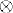# PRODUCT MEASURES

Product measures are measures defined on the product of several measurable spaces. Fubini’s theorem gives conditions under which we can evaluate integrals with respect to product measures by computing iterated integrals. In this chapter, we present the definition of product measures and Fubini’s theorem.

# 10.1 Basic Concepts and Facts

Definition 10.1 (Cartesian Product). Let S1 and S2 be two sets. The Cartesian product of S1 and S2 is a set defined asDefinition 10.2 (Measurable Rectangle). Let (S1, ∑1) and (S2, ∑2) be two measurable spaces. Let S = S1 × S2 be the Cartesian product of S1 and S2. A measurable rectangle in the product space S is a product A1 × A2 for which A11 and A22.

Definition 10.3 (Product σ-Algebra). Let (S1, ∑1) and (S2, ∑2) be two measurable spaces. The product σ-algebra ∑2 (∑12 is not a Cartesian product in the usual sense.) is defined as the σ-algebra generated by all measurable rectangles in the product space S1 × S2:

Definition ...

Get Measure, Probability, and Mathematical Finance: A Problem-Oriented Approach now with the O’Reilly learning platform.

O’Reilly members experience live online training, plus books, videos, and digital content from nearly 200 publishers.